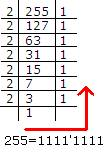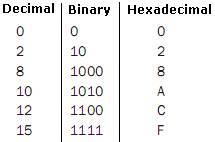Post

# Basics of Colors in XAML Silverlight

• 8.5k
• 0
• 0

Introduction

In Silverlight, many external convertors are used to develop a good application. One of the most famous here is working with colors. Converters have the task of parsing a text format of a color like '#FF0000FF' or '#0000FF' to a brush color. These are all internal processes if we are using Blend like in development environments. In Silverlight any color is defined using RGB (Red, Green, and Blue) and Alpha Channels.

While developing applications we can use any format of color either color name or its code. For example,

Red    -      #FF0000 (This is hexadecimal value with pre # sign)

Blue   -       #0000FF (This is hexadecimal value with pre # sign)

Lime   -       #00FF00 (This is hexadecimal value with pre # sign)

Green -       #007F00 (This is hexadecimal value with pre # sign)

If a color value does not have a name, then we use the code using its R, G and B values. Each R, G and B elements are coded from 0 to 255. Mixing these three (RGB) produces all colors we see and it starts from Black (0,0,0) to White (255,255,255).

We count number from 0 to 9 and 10 to  because we use the decimal system (base 10) but the computer still uses the binary system (base 2). They count with 0 and 1 and for computer's color system 0 is coded as "0000'0000" and 255 is coded as "1111'1111". Similarly, 250 is coded as "1111'1010". These results can be obtained just by finding the binary number. For example,Look at a binary and hexadecimal conversion table:Now, we can write:

255 (Decimal) = 1111'1111 (Binary) = FF (Hexadecimal)

And this is the way to represent all colors depending upon the value of RGB. For example,

White has (R=0, G=0 and B=0) so, finally its hexadecimal color code will be #000000.

Yellow has (R=255, G=255 and B=0) so, finally its hexadecimal color code will be #FFFF00.

A color without name (R=129, G=212 and B=208) will be #81D4D0.

Now in Silverlight we uses any fill color like 'Blue' will exactly be same as using #0000FF.

Be tuned for the next article.

HAVE A HAPPY CODING!!

Recommended Free Ebook
Similar Articles